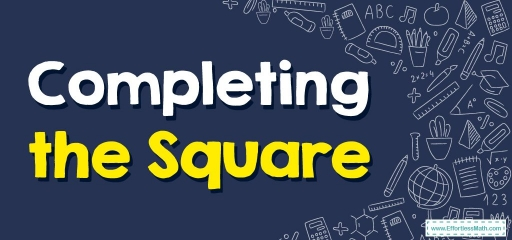# How to Solve a Quadratic Equation by Completing the Square?

Completing the square is a way used to solve a quadratic equation by changing the form of the equation. In this step-by-step guide, you learn more about the method of completing the square.When we want to convert a quadratic expression of the form $$ax^2+ bx+c$$ to the vertex form, we use the completing square method.

## A step-by-step guide to completing the square

Completing a square is a method used to convert a quadratic expression of the form $$ax^2+ bx+c$$ to the vertex form $$a(x-h)^2+k$$. The most common application of completing the square is in solving a quadratic equation. This can be done by rearranging the expression obtained after completing the square: $$a(x + m)^2+n$$, so that the left side is a perfect square trinomial.

Completing the square method is useful in the following cases:

• Converting a quadratic expression into vertex form.
• Analyzing at which point the quadratic expression has the minimum or maximum value.

### Completing the square method

The most common application of completing the square method is factorizing a quadratic equation, and henceforth finding the roots and zeros of a quadratic polynomial or a quadratic equation. We know that a quadratic equation in the form of $$ax^2+bx+c=0$$ can be solved by the factorization method. But sometimes, factorization of the quadratic expression $$ax^2+bx+c$$ is complex or impossible.

### Completing the square formula

Completing a square formula is a method for converting a quadratic polynomial or equation to a complete square with an additional constant value. A quadratic expression in variable $$x$$: $$ax^2+ bx+ c$$, where $$a, b$$ and $$c$$ are any real numbers but $$a≠0$$, can be converted into a perfect square with some additional constant by using completing the square formula or technique.

Completing the square formula is a method that can be used to find the roots of the given quadratic equations, $$ax^2+bx+c$$, where $$a, b$$ and $$c$$ are any real numbers but $$a≠0$$.

The formula for completing the square:

The formula for completing the square is:

$$\color{blue}{ax^2 + bx + c ⇒ a(x + m)^2+ n}$$

where:

• $$m$$ is any real number
• $$n$$ is a constant term

Instead of using the complicated step-by-step method to complete the square, we can use the simple formula below to complete the square. To complete the square in the expression $$ax^2+bx + c$$, first find:

$$\color{blue}{m= \frac{b}{2a}}$$ , $$\color{blue}{n=c – (\frac{b^2}{4a})}$$

Substitute these values in: $$ax^2+bx +c = a(x + m)^2+n$$. These formulas are derived geometrically.

## Exercises for Completing the Square

### Solve each equation by completing the square.

1. $$\color{blue}{x^2+12x+32=0}$$
2. $$\color{blue}{x^2-6x-3=0}$$
3. $$\color{blue}{x^2-10x+16=0}$$
4. $$\color{blue}{2x^2+7x+6=0}$$
1. $$\color{blue}{x=-4, -8}$$
2. $$\color{blue}{x=3+2\sqrt{3},\:3-2\sqrt{3}}$$
3. $$\color{blue}{x=2,8}$$
4. $$\color{blue}{x=-\frac{3}{2}, -2}$$

### What people say about "How to Solve a Quadratic Equation by Completing the Square? - Effortless Math: We Help Students Learn to LOVE Mathematics"?

No one replied yet.

X
30% OFF

Limited time only!

Save Over 30%

SAVE $5 It was$16.99 now it is \$11.99